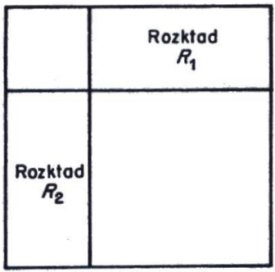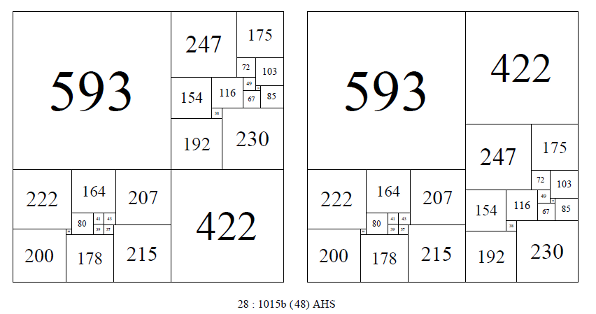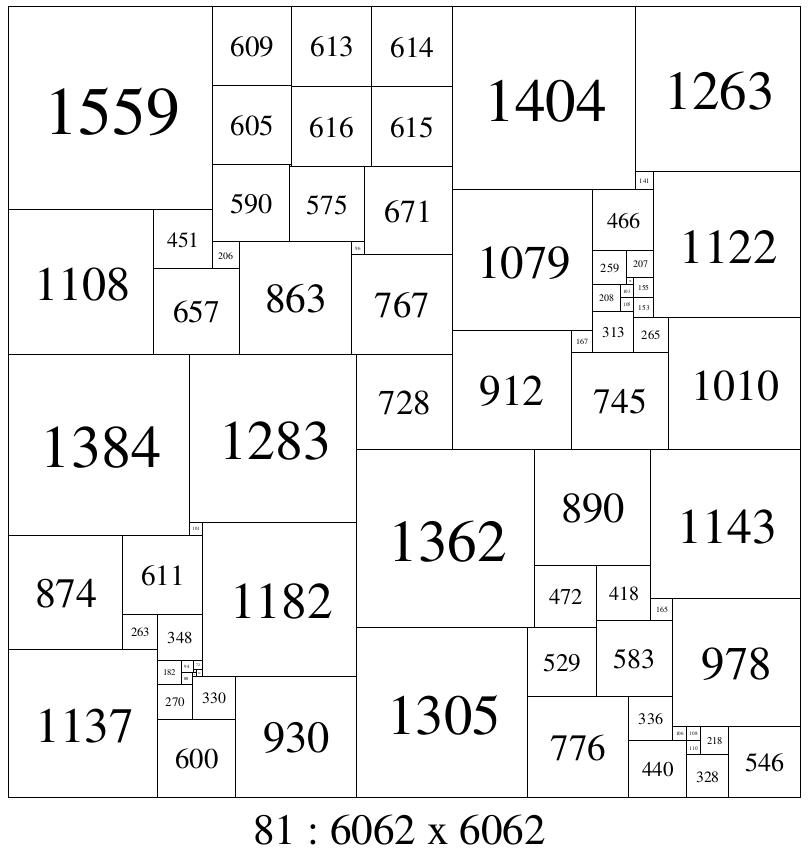# Simple Perfect Squared Rectangles and Disjoint n-tuples

If A and B are two sets and the intersection of the elements of A and B is the empty set; A ∩ B = ∅, then we can say the elements of A and B are disjoint. If A and B are two Squared Rectangles or two Squared Squares with no element in common, then we can call such things disjoint n-tuples. We could call such A and B disjoint pairs but we can extend the meaning to cover A,B,C triples, A, B, C, D quadruples etc if we call them disjoint n-tuples. Perfect Squared Squares (PSSs) A and B can be compared to each other by their element sizes, as can SPSRs A and B of the same shape. If A and B are rectangles, and are the same shape then the height and width of the rectangles are in the same ratio, but they may not be the same size, if this is the case, then we can scale A and B to the same sizes before comparing elements to see if they are disjoint.

The reason we may want to seek out disjoint n-tuples (with SPSRs in particular) is that they provide the necessary ingredients of a well known method for constructing Compound Perfect Squared Squares (CPSSs).Moroń's figure

In 1925 Zbigniew Moroń  raised the question “For what squares is it possible to dissect them into squares?” He then observes “if there exists a rectangle (of different sides) for which there are two dissections R1 and R2 such that; in neither of these dissections does there appear a square equal to to the smaller side of the rectangle and, each square of dissection R1 is different from each square in dissection R2, then the square is dissected into squares, all different, as shown in the following figure.”

In 1940 Arthur Stone  published the discovery of a CPSS of side 1015 and order 28 using the Moroń construction. It was constructed from 2 disjoint n-tuple SPSRs of order 13, augmented with two additional squares, equal in size to the respective side lengths of the rectangles. Order 13 is the lowest order in which such disjoint n-tuples appear. Disjoint n-tuple SPSRs of the same size appear in all higher orders of SPSRs in exponentially increasing numbers. From orders 13 to 21, the number of same size disjoint SPSR pairs are 1, 2, 7, 60, 225, 1208, 5186, 20116, 82227. From these pairs an equivalent number of CPSSs can be constructed. In fact even more CPSSs can be created; if 3 SPSRs are a disjoint triple then 3 CPSSs can be produced rather than just two pairs of CPSSs and similarly 6 CPSS can be produced by using 2 pairs at a time from disjoint quadruple SPSRs and so on. Yet even more CPSS can be produced from disjoint quadruples by using all four SPSRs at once. Additional CPSSs can also be produced by comparing SPSRs across orders and by comparing SPSRs of the same shape. The order of the CPSSs produced with 2 SPSRs is double the order of the SPSRs plus 2.Two of the 48 isomers of 28: 1015b. Arthur Stone (1940)

Each CPSS created from a disjoint pair in this manner can be arranged in 48 isomer classes. Isomers are CPSSs with the same elements arranged differently. Trivial isomerisms such as rotating or reflecting the whole CPSS as a rigid block are not included. The 48 isomer classes can be split into 2 subclasses of 16 and 32, where the two rectangles can be arranged by rotation and reflection in place and also swaps with each other.

A special case exists where two 1x2 SPSRs (of the same size, or scaled to the same size) can be combined along the long side to form a CPSS without the addition of the two extra squares. Three 1x3 SPSRs can be similarly combined to form a CPSS (but only 1 1x3 SPSR is known)

In 1990 Jasper Skinner  reported the existence of two unique compound perfect dissections of a square of reduced side 1429 into 29 unequal squares. He wrote, 'To be termed “uniquely squared” two or more perfect squared squares (or rectangles) must meet three criteria: (1) be of the same order, (2) have equivalent corresponding reduced sides, and (3) have no element in common. Many pairs of perfectly squared squares are known but have common elements. Pairs of uniquely squared simple perfect rectangles of common reduced sides and order first appear at order 13. The low order pair of simple perfect rectangles was completely described by A. H. Stone (1940) [ 3] Triplets of common reduced sides and order first appear at order 16 as indicated by C. J. Bouwkamp (1965) . Special thanks are extended to C. J. Bouwkamp for permission to publish his triplet discovery. The Bouwkampcode of the low order triplets of reduced side ratio 2393:3218 reads as follows:
(A) (1242,627,548, 801) (79,469) (706) (216,585) (316,369) (1151,91) (1060, 53) (1007);
(B) (1243, 1091,884) (207,677)(245,583,470)(1150,93)(338) (315,832)(719,202)(517);
(C) (1273,708, 1237) (312,396) (228,84) (347, 133) (214, 1156) (25,203) (I 120, 178) (942).
At present, no quadruplet example of uniquely squared simple perfect rectangles of common reduced sides and order is known. However, C. J. Bouwkamp (1965) has discovered a pair of quadruplets with no common element though the reduced sides differ and the order ranges from 17 to 18."

### Disjoint n-tuple Squared Squares

Skinner constructed the first pair of uniquely squared squares of common reduced side and order. "These dissections were discovered empirically on May 4-6, 1990 using a technique of T. H. Willcocks (1951) [5, Technique 2.211 applied to two compound perfect squares of 28th-order: the first of reduced side 1015 due to A. H. Stone (1940)  and the second of reduced side 1073 described by W. T. Tutte (1950) . The result was a pair of 29th-order compound perfect squares of reduced side 1429 with no common element. The Bouwkampcode of the first reads as follows:
(414,280, 372, 363)(188,92) (93,270) (119,261,84) (199,215) (177) (165,23) (142) (183, 16)(167,47,17) (13,43, 163,796) (30) (120) (633).
The Bouwkampcode of the second is;
(356,244, 153,248,169,259)(91,62) (79,90) (29,33) (364) (360)(349) (111,89, 156) (22,67) (133) (88, 135) (221) (39, 213,821) (174)(608)."

### Squared Windmill

As alluded to above, if one has four disjoint n-tuple SPSRs then a variety of new CPSS constructions are possible. These are extensions of the Moroń construction and its isomers along with a new construction, the Squared Windmill. A squared windmill has 4 rectangles arranged around a central square (equal in size to the difference of the width and height of the rectangles), rather like a windmill.

It is not known what is the lowest order appearance of quadruple disjoint n-tuple SPSRs in the same order. However it is known (Anderson 2011) that a quadruple of disjoint n-tuple SPSRs exists in order 20. A windmill has been created from this quadruple;Squared Windmill, o81 : 6062 SEA (2012)

### References

1. 'O Rozkladach Prostokatow Na Kwadraty' (On the Dissection of a Rectangle into Squares) by Zbigniew Moroń, Prezeglad Mat. Fiz. 3 152-153 (1925)
2. R.L. Brooks, C.A.B. Smith, A.H. Stone and W. T. Tutte. 'The Dissection of Rectangles into Squares'. Duke Math. J. 7 (1940) 312–340.
3. E401 A. H. Stone, Michael Goldberg and W. T. Tutte, The American Mathematical Monthly Vol. 47, No. 8 (Oct., 1940), pp. 570-572
4. JASPER DALE SKINNER II, 'Uniquely Squared Squares of a Common Reduced Side and Order', JOURNAL OF COMBINATORIAL THEORY, Series B 54, 155-156 (1992)
5. T. H. WILLCOCKS, A note on some perfect squared squares, Canad. J. Math. 3 (1951),304-308.
6. W. T. TUTTE, Squaring the square, Canad. J. Math. 2 (1950),197-209.